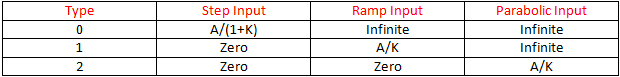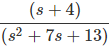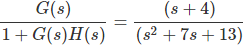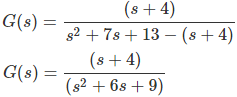# MCQs on Control Systems

##### Page 5 of 23. Go to page 1 2 3 4 5 6 7 8 9 10 11 12 13 14 15 16 17 18 19 20 21 22 23
01․ What is the order of a closed loop control system for a plant with a transfer function equal to G(s) = k/s²(1+Ts) and with unity feedback
3
2
1
0

Every transfer function representing a control system has certain type and order. The transient state analysis depends on the order of the control system. The order of system is obtained from closed loop transfer function. The highest power of the characteristic equation (1 + G(s)H(s) )determines the order of the control system. For given problem, characteristic equation is 1 + G(s)H(s) = 0 s²(1+Ts)+k = 0 Therefore, order = 3

02․ A second order control system is defined by the following equation: 4 d²c(t)/dt² + 8 dc(t)/dt + 16 c(t) = 16 u(t) The damping ratio and natural frequency for this system are respectively

The standard second order expression in terms of damping ratio (ξ) and natural frequency (ωn) is as follows. C(s)/R(s) = (ω²n)/(s² + 2ζ(ωn)s + ω²n) Given second order system is C(s)/U(s) = 16/( 4 s²+ 8 s + 16) = 4/(s² + 2 s + 4) Therefore, comparing above two equations, Natural frequency = 2 rad/s Damping ratio = 0.5

03․ The steady state error due to ramp input for a type two system is equal to
zero.
infinite.
non zero number.
constant.

Error equation E(s) = R(s)/(1 + G(s) H(s) ) Applying final value theorem, Steady state error = Lts→0 S E(s) Steady state error for different types and different inputs as follows:04․ Differentiation of parabolic response is a -------------- response?
parabolic
ramp
step
impulse

Parabolic response r(t) = A* t²/2 Differentiation of parabolic response is dr(t)/dt = A*t Therefore, differentiation of parabolic response gives the ramp response. d(Parabolic response)/dt = Ramp response d(Ramp response)/dt = Step response d(step response)/dt = Impulse response

05․ Weighting function is in terms of -----------------
Laplace transform of the step response
Laplace transform of the ramp response
Laplace transform of the parabolic response
Laplace transform of the impulse response

d(Parabolic response)/dt = Ramp response d(Ramp response)/dt = Step response d(step response)/dt = Impulse response L(impulse response) = Transfer function The transfer function in terms of Laplace transform of impulse response is known as weighting function.

06․ The impulse response of a system is c(t) = -te-t + 2 e-t (t>0). Its closed loop transfer function is
(2s+1)/s²
(2s+1)/(s+1)²
(2s+2)/(s+1)²
(2s+2)/s²

L(Impulse response) = Transfer function C(s) = -1/(s+1)² + 2/(s+1) Closed loop transfer function = (2s+1)/(s+1)²

07․ What is the open loop DC gain of a unity feedback control system having closed loop transfer function as4/13
13/4
9/4
4/9

Closed loop transfer function =Given system is a unity feedback control system, Open loop transfer functionFor DC gain AC terms should be zero, i.e., s = 0 Therefore, open loop DC gain G(s) = 4/9.

08․ The impulse response of a system is c(t) = -te-t + 2 e-t (t>0). Its open loop transfer function is
(2s+1)/s²
(2s+1)/(s+1)²
(2s+2)/(s+1)²
(2s+2)/s²

L(Impulse response) = Transfer function C(S) = Transfer function = (2s+1)/(s+1)² G(s)/(1 + G(s) H(s) ) = (2s+1)/(s+1)² Open loop transfer function G(S) = (2s+1)/((s+1)² - (2s+1)) G(s) = (2s+1)/s²

09․ Certain control system has input u(t) and output c(t). If the input is first passed through a block having transfer function e-s and unit gain is applied to the applied to the system. The modified output will be
c(t-1).u(t)
c(t-1).u(t-1)
c(t).u(t-1)
c(t)u(t)

Let F(s) is block gain, then C(s) = F(s).R(s) If the input passed through a block having transfer function e-s Cm(s) = R(s).e-s.F(s) Cm(s) = C(s).e-s By taking inverse Laplace transforms Cm(t) = c(t-1).u(t-1)

10․ Lead compensator is used to improve ------------------
Both 1 and 2
None of the above

Compensators in control system are used to improve the performance specifications, i.e. transient and steady state characteristics.
1. Lead Compensator: It is used for improving the transient state or speed response of the system.
2. Lag Compensator: It is used for improving steady state response characteristics of the system, i.e elimination of steady state error between output and input.
3. Lag-Lead or Lead-Lag Compensator: It improves both transient and steady state response characteristics. It exhibits both lead and lag characteristics in its frequency response.

<<<34567>>>